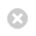Close

UCL Centre for Languages & International Education (CLIE)

Home# Mathematics (UPCH)

Find out more about the Mathematics foundation subject on the UPCH. Learn about what you will study, teaching methods, assessments and recommended reading.

## Key information

Subject title: Mathematics

Subject type: optional

UPC Pathway: UPCH

Number of students (2023-24): 35

## Subject description

The Mathematics foundation subject is an optional part of the Undergraduate Preparatory Certificate for Humanities (UPCH) course. You’ll gain an in-depth coverage of topics usually taught in A-level Mathematics and Further Mathematics.

The Mathematics foundation subject will prepare you thoroughly with the skills you need to study at UK universities. This subject's students usually go on to study degrees such as Economics BSc, Economics and Statistics BSc, Mathematics BSc and Art and Sciences BASc.

The syllabus covers the following topics:

### Differentiation

• Differentiation from first principles
• Derivatives of polynomial, trigonometric, hyperbolic, exponential and logarithmic functions, parametric equations
• The chain rule, product rule and quotient rule
• Implicit differentiation
• Tangent and normal lines
• Stationary points and curve sketching
• Taylor series and Maclaurin series

### Integration

• Indefinite integrals
• Integration techniques: substitution, integration by parts, trigonometric and hyperbolic substitution
• Definite integrals
• Applications to area and volume of revolution
• Separable differential equations

### Algebra and Hyperbolic Functions

• Quadratic equations and their roots. Sum and product of roots
• The remainder and factor theorems
• The generalised binomial theorem for all real powers, with applications
• Hyperbolic functions and their inverses and calculus

### Series

• Convergence and divergence
• Arithmetic and geometric sequences and series
• Tests for convergence: nth term test, comparison test, limit comparison test, ratio test
• Compound interest. Applications to investments and mortgages
• Proof by induction

### Complex Numbers

• Basic arithmetic and notation
• Argand diagrams
• Polar form
• de Moivre’s Theorem and applications
• Complex roots of numbers
• Exponential form
• Loci

### Matrices

• Matrix arithmetic
• Inverse matrices
• Systems of linear equations, Gaussian elimination and echelon forms

### Probability

• Counting: permutations and combinations, distinct and identical objects
• Probability: and, or and conditional probability
• Discrete random variables. Mean, standard deviation and variance
• The binomial distribution
• Continuous random variables. Mean, standard deviation, variance and mode

## How we teach Mathematics

Each week you’ll have two in-person lectures and attend two small-group tutorials. In these classes, you’ll work on mathematics exercises and activities based on the lectures.

We expect you to carry out independent study and problem-solving outside of your classes.

Office hours will be available for additional support.

## Intended learning outcomes

By the end of the subject, you should be able to:

• state and prove the main theoretical results the syllabus is based on
• apply the theory to problem-solving
• present well-written solutions to problems with good attention to detail and a logical structure
• work independently on mathematics exercises, taking responsibility for your own understanding and asking for help when necessary
• confidently discuss mathematical ideas in English, using correct vocabulary

## Overview of Summative Assessment

TermAssessmentWeighting
OneTest (2 hours)10%
TwoTest (2 hours)10%
ThreeFinal Exam (3 hours)60%
Throughout the yearClass Tests (50 mins each)10%
Coursework Assignments10%
100% total

Assessment weighting is for the 2022-23 academic year. This may change for 2023-24 entry.

Before starting the course, you should prepare by revising high school work.

You’ll need to be comfortable with logarithms and have strong trigonometry knowledge. You should be able to work with all six trigonometric functions (sine, cosine, tangent, cotangent, secant, cosecant) and the six inverse trigonometric functions. You should also be familiar with standard trigonometric identities and using them in proofs.

Any standard high school mathematics text should cover this material.

The set text (Mathematics - The Core Course for A-level, by L Bostock and S Chandler, published by Stanley Thornes, ISBN 0859503062) covers this material in chapters six and seven.

All students should buy the set course textbooks and calculator.

• Calculator: Casio fx-83GT X, cost is approximately £10. (Older models are acceptable.)

• Textbooks: 'Mathematics- The Core Course for A-level’, by L Bostock and S Chandler, published by Stanley Thornes, ISBN 0859503062. This book costs approximately £35, if bought new. Cheaper second-hand copies are available.

• Further Pure Mathematics’, by L Bostock, S Chandler and C Rourke, published by Stanley Thornes, ISBN 0859501035. This also costs around £35 if bought new.

## Subject selection guide

Please check out our optional subject selection guide for information on suitable subjects for your future degree plans.

Please note the information on all the UPC subject pages, including this one, reflects the subject as it was taught in the 2022-23 academic year (unless otherwise stated).